Processing ......FreeComputerBooks.com Links to Free Computer, Mathematics, Technical Books all over the World

Applied Probability
🌠 Top Free Java Books - 100% Free or Open Source!
• Title: Applied Probability
• Author(s) Paul Pfeiffer
• Publisher: OpenStax-CNX (2020); eBook (Creative Commons Licensed)
• Hardcover/Paperback: N/A
• eBook: HTML, PDF (634 pages), ePub, Kindle, etc.
• Language: English
• ISBN-10/ASIN: N/A
• ISBN-13: N/A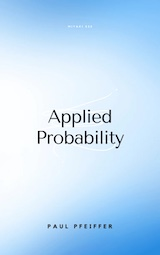Book Description

This book presents a unique blend of theory and applications, with special emphasis on mathematical modeling, computational techniques, and examples from the real world application in industries and science.

This is a "first course" in the sense that it presumes no previous course in probability. The mathematical prerequisites are ordinary calculus and the elements of matrix algebra. A few standard series and integrals are used, and double integrals are evaluated as iterated integrals. The reader who can evaluate simple integrals can learn quickly from the examples how to deal with the iterated integrals used in the theory of expectation and conditional expectation. Appendix B provides a convenient compendium of mathematical facts used frequently in this work.

Reviews, Rating, and Recommendations: Related Book Categories: Read and Download Links:Similar Books:
•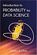Introduction to Probability for Data Science (Stanley Chan)

This book is an introductory textbook in undergraduate probability in the context of data science to emphasize the inseparability between data (computing) and probability (theory) in our time, with examples in both MATLAB and Python.

•Introduction to Probability, Statistics, and Random Processes

This book introduces students to probability, statistics, and stochastic processes. It provides a clear and intuitive approach to these topics while maintaining mathematical accuracy. The book contains a large number of solved exercises.

•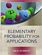Elementary Probability for Applications (Rick Durrett)

This clear and lively introduction to probability theory concentrates on the results that are the most useful for applications, including combinatorial probability and Markov chains. Concise and focused, it is designed for students familiar with basic calculus.

•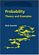Probability: Theory and Examples (Rick Durrett)

This lively introduction to measure-theoretic probability theory covers laws of large numbers, central limit theorems, random walks, martingales, Markov chains, ergodic theorems, and Brownian motion.

•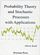Probability Theory and Stochastic Processes with Applications

This book provides an introduction to probability theory and discrete and continuous stochastic processes and its applications. It has a unique approach that provides a broad and wide introduction into the fascinating area of probability theory.

•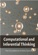Computational and Inferential: The Foundations of Data Science

Step by step, you'll learn how to leverage algorithmic thinking and the power of code, gain intuition about the power and limitations of current machine learning methods, and effectively apply them to real business problems.

•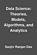Data Science: Theories, Models, Algorithms, and Analytics

It provides a bucket full of information regarding Data Science, covers a wide variety of sections by giving access to theories, data science algorithms, tools and analytics. You'll explore the right approach to best practices to guide you along the way.

•Python Data Science Handbook: Essential Tools (Jake VanderPlas)

Several resources exist for individual pieces of this data science stack, but only with the Python Data Science Handbook do you get them all - IPython, NumPy, Pandas, Matplotlib, Scikit-Learn, and other related tools.

•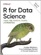R for Data Science: Visualize, Model, Transform, Tidy, Import

This book teaches you how to do data science with R: You'll learn how to get your data into R, get it into the most useful structure, transform it, visualize it and model it, how data science can help you work with the uncertainty and capture the opportunities.

Book Categories
 :All CategoriesTop Free BooksRecent BooksMiscellaneous BooksComputer EngineeringComputer LanguagesComputer ScienceData Science/DatabasesJava and Java EE (J2EE)Linux and UnixMathematicsMicrosoft and .NETMobile ComputingNetworking and CommunicationsSoftware EngineeringSpecial TopicsWeb Programming
Other Categories